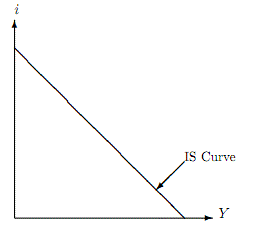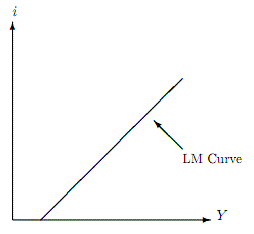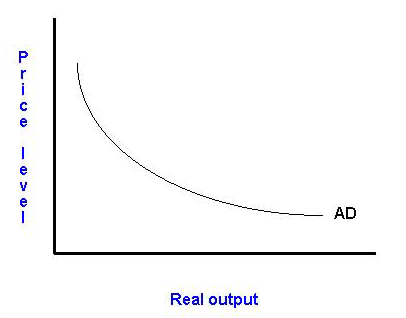### Seeing is believing!

Before you order, simply sign up for a free user account and in seconds you'll be experiencing the best in CFA exam preparation.

##### Subject 3. Aggregate Demand
Aggregate demand (AD) is the quantity of goods and services that households, businesses, and foreign customers want to buy at any given level of prices.

The IS Curve

GDP = C + I + G + (X - M)

• Consumption (C) is a function of disposable income. Consumption increases when income(Y) increases and/or taxes decrease.

The marginal propensity to consume (MPC) is defined as additional current consumption divided by additional current disposable income. The marginal propensity to save (MPS) = 1 - MPC.

• Investment spending depends on the interest rate (i) and output/income level.

• Government purchases are assumed to be unrelated to both interest rates (i) and income (Y). Although tax policy is also considered an exogeneous variable, the actual taxes collected depend on income (Y) and are therefore endogenous. The government's deficit (G - T) increases as income decreases and vice versa.

• Net exports (X - M) depends on relative income and prices between the domestic country and the rest of the world.

We can also derive the following equation, which shows that domestic saving has three uses: investment, government deficits, and trade surplus:

S = I + (G - T) + (X - M), where S is domestic saving.

If we combine these relationships together we can derive the IS curve: the combination of GDP (Y) and the real interest rate (i) such that aggregate income/output equals planned expenditures.Note that there is an inverse relationship between income and the real interest rate. For example, when interest rates are high, investment falls and therefore Y must fall as well.

Note that changes in Y caused by changes in i are reflected as movements along the IS curve. On the other hand, changes in Y that are brought about by factors other than interest rates will cause Y to change, regardless of the level of interest rates in the economy. For example, changes in government purchases will not change the slope but will change the intercepts; in other words, they will cause the IS curve to shift.The LM Curve

The IS curve depicts combinations of interest rates and output that clears markets for goods and services. The IS curve by itself does not pin down the interest rate that prevails in the economy. In order to do so, we look at the money market. The LM curve summarizes all the combinations of income and interest rates that equate money demand and money supply.

The quantity theory of money: MV = PY, where V is the velocity of money.

• When interest rates (i) are high, the demand for money is low because money pays no interest; the opportunity cost of holding money rises.
• When Y is high, the demand for money is high; richer people buy more goods and are likely to hold more money.
• When P is hig,h the demand for money is high because we need more money to buy goods.

When the money market is in equilibrium, money supply = money demand. The LM curve summarizes all the combinations of income and interest rates that equate money demand and money supply. It is an upward-sloping relationship between i and Y.Intuitively, we can explain the upward-sloping LM curve as follows: Let's consider some combination of income and interest rates that equates money demand with the money supply set by the Fed. Now suppose there is an increase in income. The increase in income causes the demand for money to increase. However, money supply is unaffected by the increase in income. The only way that money demand and money supply can be equal again is if interest rates also increase to reduce money demand.

The Aggregate Demand Curve

Combining the IS and LM relationships yields the aggregate demand curve, which depicts the inverse relationship between the price level and real income/output, assuming a constant money supply.For example, a reduction in the price level will:

• Increase the wealth of people holding the fixed quantity of money.
• Reduce the real rate of interest.
• Make domestically produced goods cheaper than those produced abroad.

All these factors will lead to an increase in the quantity of goods and services demanded at the lower price level (movement along the curve).

Learning Outcome Statements

e. explain the fundamental relationship among saving, investment, the fiscal balance, and the trade balance;

f. explain the IS and LM curves and how they combine to generate the aggregate demand curve;

CFA® 2020 Level I Curriculum, 2020, Volume 2, Reading 14

User Comment
achu Increased foreign income & DECREASED exchange rate , both spur export demand.
Mijalko Did not like the explanation for IS curve so I'll post my own. Real interest rate (i) can be seen as difference between S and I. The lower the i the smaller S-I as less people will save and more people will invest. The more they invest the more goods are produced so the less the interest rate the higher the output which explains the inverse curve.
Mijalko Having read LM I do not understand one thing. If Y is high, than interest rate should be high in order to keep real money supply constant. But from IS perspective, high interest rate leads to low investment and low Y. So on one side high interest rate leads to decrease of Y and on the other side it is neccessary when Y is high. It's a bit confusing. Anyone?
olympria I think this would answer Mijalko
This is what I pictured it as:

The Y in the IS curve depicts the Income side of GDP
The Y in the LM curve depicts the Output side of GDP (both should be the same anyway)
The IS curve acts like the demand curve -> The LM curve acts like the supply curve } both related to 2 factors - interest rates and Y(income/output) instead on Price and Qty in the usual demand/supply curves
Therefore, if you place both curves one on top of the other, the intersection will be the prevailing interest rate and output/income.

Please correct me if I am wrong.
fobucina If we look at the money-market (where y-axis is r and x-axis is M/P), a higher real output (Y) would initially shift the money demanded to the right (as more individuals look to increase their liquidity due to increased transactional benefits). This initial imbalance between money demanded and the real money supply would require the real interest rates of securities to increase in order for equilibrium in the money market to be restored (i.e., the opportunity cost of holding money increases). As a result, the higher interest rates will lower investment and consumption and, in turn, reduce real output on the IS curve. Therefore, I believe the decrease in Y on the IS curve proceeds the events that play out in the money market.
sshetty2 Something that confused me ad nauseam when i was going through this the first time was why investment spending would go down when interest rates went down. I mean wouldn't you want to invest more if you would earn more on your money?.. The way I like to think about the interest rate now is that it is not the interest rate you would earn on your money, but instead, the risk-free required rate of return on investments. If this goes up then investment spending will go down and vice-versa because the required rate of return will make some investments unfeasible.